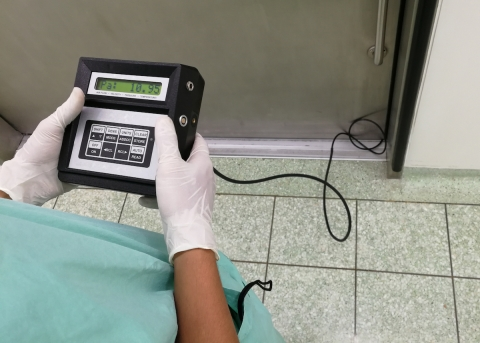# Testing a Smoke Control Boundary

Posted by: Robert Cave on Thursday, September 26, 2019One Newton per square meter.

This is a familiar term to some engineers but who remember what it means? To impress your listeners, mention that an acceptable pressure drop across a smoke barrier in a full sprinkled building is 12.5 Newton/square meter.

So, what is a Newton/square meter? That is too much to go into with this blog when all we need to know one Newton/square meter is equal to the U.S. customary unit of one Pascal.

Now we are talking a language common to most engineers because we know what a Pascal is. Right? A pascal is a measurement of pressure.

To be precise, a Pascal is 1/249th of an inch of water. That means cutting a column of water one inch deep into 249 equal pieces. The pressure below one of those very thin columns of water is equal to one Pascal (Newton/square meter) or 4.02x10-3 PSI.

A very small number.

When testing smoke barriers, a pressure drop of 12.5 Pascal (Newton/square meter) is enough to keep smoke from crossing this boundary. In addition, 12.5 Pascal is a measurable pressure using today’s sophisticated equipment.

So, the next time you have to test a smoke barrier you can specify the minimum required pressure drop in Newton/square meter or Pascal to be more precise.